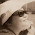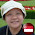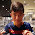## Wednesday, 17 February 2016

### Algebra... Different "Faces" of Algebra (I)

The total length of 4 roads is (3x - 1) km.
What are the possible lengths of the 4 roads in kilometres?
Note that x is an integer.

e.g. The length of the 4 roads are: x km, x km, x-7 km, 6 km
Check: x + x + (x - 7) + 6 = 3x - 1

You may also enter any assumptions you made to your answers.

1.The length of the 4 roads are:
x-5 km, x km, x km, 4 km
Check: x + x + x-5 + 4 = 3x-1

1.You are correct.
However, you can be specific, what assumption(s) did you make about the value of x?

2.The possible lengths of the 4 roads are:
Xcm
(X-10)cm
(X+5)cm
4cm
Check:(X+(X-10)+(X+5)+4)cm =3x-1

1.Didn't she say km? Not cm...

2.You are correct.
However, you can be specific, what assumption(s) did you make about the value of x?

3.The assumptions I made were
X is a positive integer
X is larger than 10

3.The possible lengths are:
Xkm
(X-8)km
7km
Xkm
check:
X+X+X+7-8=(3X-1)km

1.You are correct.
However, you can be specific, what assumption(s) did you make about the value of x?

2.I assumed that x is greater than 8

4.Possible lengths:
x km
x km
(x-2) km
1 km
Check: x+x+x-2+1=(3x-1) km

5.This comment has been removed by the author.

1.Check:
Are the proposed measurements valid?
What's the assumption?

6.x-3,x-8,x and 10km
Check:3x-(-11)+10=3x(-1)km

1.You are correct.
However, you can be specific, what assumption(s) did you make about the value of x?

7.The lengths can be
1)x
2)x-4
3)x-6
4)9
Check: (x+(x-4)+(x-6)+9=3x(-1)

1.2.This comment has been removed by the author.

3.You are correct.
However, you can be specific, what assumption(s) did you make about the value of x?

4.x is bigger than 7

5.6.8.The lengths of the roads may be (in km)
1)x-1
2)x-2
3)x-3
4)5

1.You are correct.
However, you can be specific, what assumption(s) did you make about the value of x?

9.The possible lengths of the 4 roads are:
1) (x-9) km
2) (x+4) km
3) (x+2) km
4) 2km
Check: ((x-9)+(x+4)+(x+2)+2)km= (3x-1)km

1.You are correct.
However, you can be specific, what assumption(s) did you make about the value of x?

10.1)X
2)X
3)X+10
4)9km
total= 3x-1

1.Correct.
What condition(s) do you need to include for the answer?

2.I assume that x is a positive integer and is greater than 7.

11.Possible length of roads:
1) (X-2)km
2) (X-2)km
3) (X-1)km
4) 4km

Check: (3X-5+4)km = (3X-1)km

1.2.However, you can be specific, what assumption(s) did you make about the value of x?

12.Possible lengths: x km, (1x-10)km, 9km, x km
Check: 9 + x + x +(x-10)= (3x -1)km

1.Check:
Are the proposed measurements valid?
What's the assumption?

2.Assuming x is a positive integer and it is greater than 7

13.Possible lengths: x km, (1x-10)km, 9km, x km
Check: 9 + x + x +(x-10)= (3x -1)km

1.You are correct;
However, you can be specific, what assumption(s) did you make about the value of x?

14.15.16.Possible Lengths:
0.75+0.25

Note: only a member of this blog may post a comment.